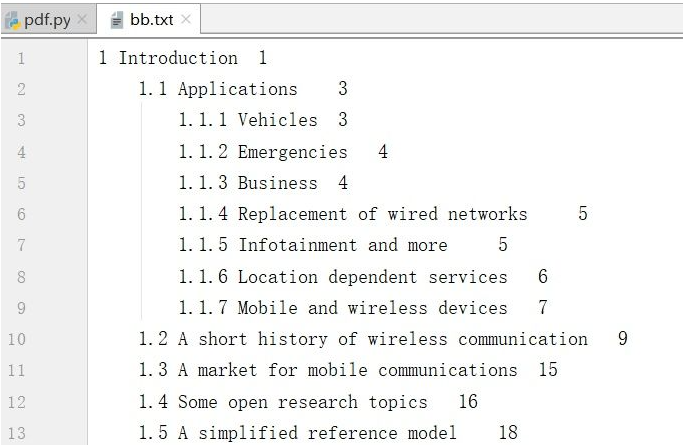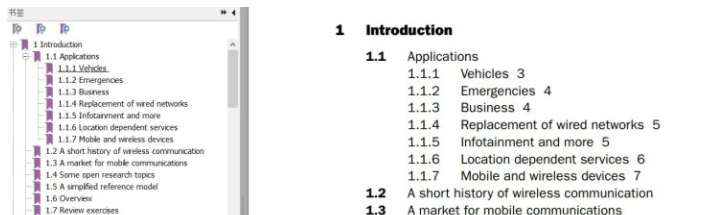• 写文章
• 提问

# Python生成pdf目录书签的方法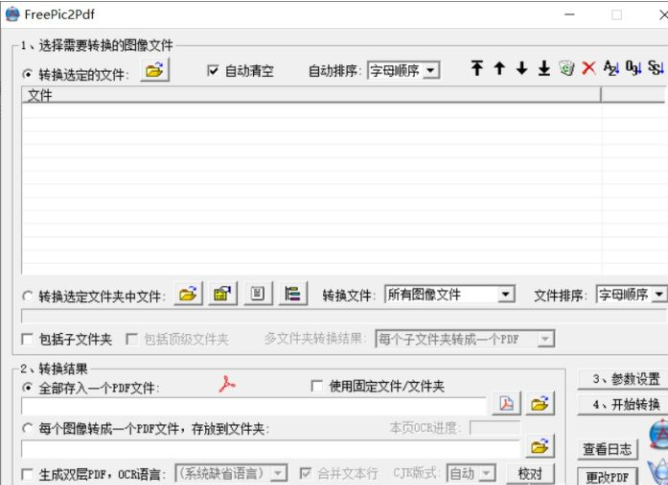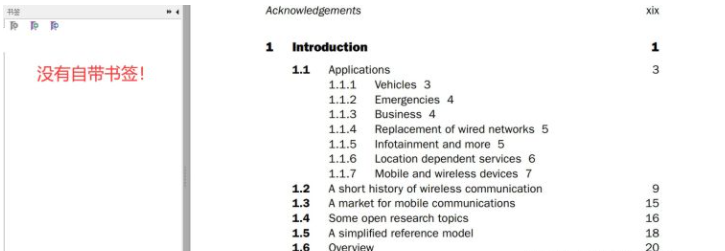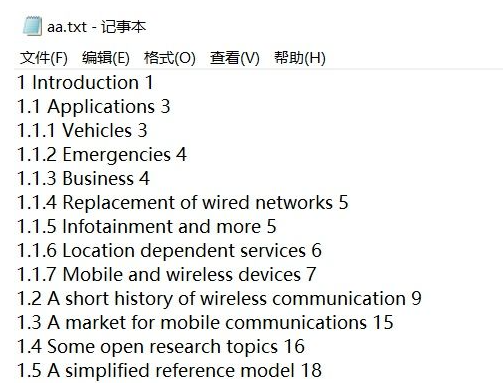```# 编码格式需要txt保存的编码格式是什么，只要最终文件不乱码就ok
fin = open("aa.txt", "r", encoding='GBK')
fout = open("bb.txt", "w")

for each_line in fin:
list = each_line.split()
for i in range(len(list) - 1): #倒数第一个元素是数字，先不放
index=list[i].split('.')
if(len(index)>1):
# 第二级目录
fout.write('\t')
if(len(index)>2):
# 第三级目录
fout.write('\t')
# 假如还有下级目录，可以继续追加...
#  输出序号
fout.write(list[i])
# 输出空格
fout.write(' ')
# 为书页号置tap位
fout.write('\t')
num = int(list[-1]) + 0 #单独处理倒数第一个数字
fout.write(str(num))#书页号
# 下一行
fout.write("\n")

fin.close()
fout.close()```